# Algebra 1 : How to solve two-step equations

## Example Questions

1 2 23 24 25 26 27 28 29 31 Next →

### Example Question #301 : How To Solve Two Step Equations

Solve for.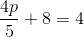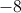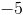Explanation:Subtract 8 from each side of the equation.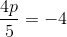Multiply each side of the equation by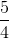(the inverse of).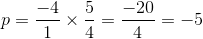### Example Question #302 : How To Solve Two Step Equations

Solve for.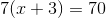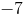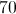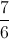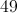Explanation:Distribute 7 to the term in parentheses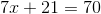Subtract 21 from each side of the equation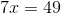Divide each side by 7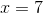1 2 23 24 25 26 27 28 29 31 Next →

### All Algebra 1 Resources